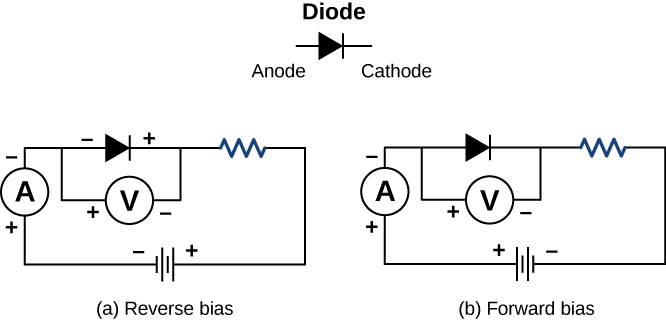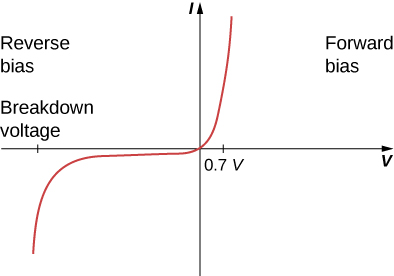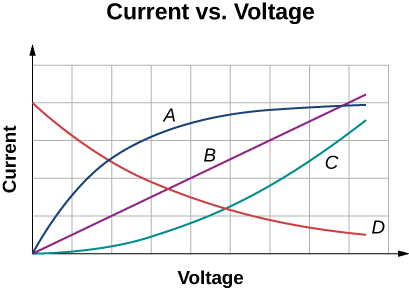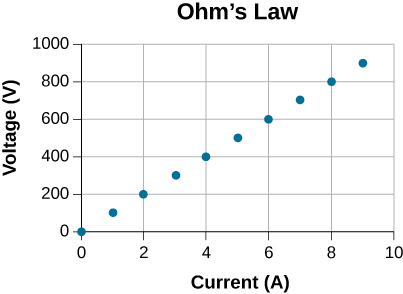# 9.4 Ohm's law  (Page 3/7)

 Page 3 / 7A diode is a semiconducting device that allows current flow only if the diode is forward biased, which means that the anode is positive and the cathode is negative.

A plot of current versus voltage is shown in [link] . Note that the behavior of the diode is shown as current versus voltage, whereas the resistor operation was shown as voltage versus current. A diode consists of an anode and a cathode. When the anode is at a negative potential and the cathode is at a positive potential, as shown in part (a), the diode is said to have reverse bias. With reverse bias, the diode has an extremely large resistance and there is very little current flow—essentially zero current—through the diode and the resistor. As the voltage applied to the circuit increases, the current remains essentially zero, until the voltage reaches the breakdown voltage and the diode conducts current, as shown in [link] . When the battery and the potential across the diode are reversed, making the anode positive and the cathode negative, the diode conducts and current flows through the diode if the voltage is greater than 0.7 V. The resistance of the diode is close to zero. (This is the reason for the resistor in the circuit; if it were not there, the current would become very large.) You can see from the graph in [link] that the voltage and the current do not have a linear relationship. Thus, the diode is an example of a nonohmic device.When the voltage across the diode is negative and small, there is very little current flow through the diode. As the voltage reaches the breakdown voltage, the diode conducts. When the voltage across the diode is positive and greater than 0.7 V (the actual voltage value depends on the diode), the diode conducts. As the voltage applied increases, the current through the diode increases, but the voltage across the diode remains approximately 0.7 V.

Ohm’s law is commonly stated as $V=IR$ , but originally it was stated as a microscopic view, in terms of the current density, the conductivity, and the electrical field. This microscopic view suggests the proportionality $V\propto I$ comes from the drift velocity of the free electrons in the metal that results from an applied electrical field. As stated earlier, the current density is proportional to the applied electrical field. The reformulation of Ohm’s law is credited to Gustav Kirchhoff, whose name we will see again in the next chapter.

## Summary

• Ohm’s law is an empirical relationship for current, voltage, and resistance for some common types of circuit elements, including resistors. It does not apply to other devices, such as diodes.
• One statement of Ohm’s law gives the relationship among current I , voltage V , and resistance R in a simple circuit as $V=IR$ .
• Another statement of Ohm’s law, on a microscopic level, is $J=\sigma E$ .

## Conceptual questions

In Determining Field from Potential , resistance was defined as $R\equiv \frac{V}{I}$ . In this section, we presented Ohm’s law, which is commonly expressed as $V=IR$ . The equations look exactly alike. What is the difference between Ohm’s law and the definition of resistance?

Shown below are the results of an experiment where four devices were connected across a variable voltage source. The voltage is increased and the current is measured. Which device, if any, is an ohmic device?Device B shows a linear relationship and the device is ohmic.

The current I is measured through a sample of an ohmic material as a voltage V is applied. (a) What is the current when the voltage is doubled to 2 V (assume the change in temperature of the material is negligible)? (b) What is the voltage applied is the current measured is 0.2 I (assume the change in temperature of the material is negligible)? What will happen to the current if the material if the voltage remains constant, but the temperature of the material increases significantly?

## Problems

A $2.2\text{-k}\phantom{\rule{0.2em}{0ex}}\text{Ω}$ resistor is connected across a D cell battery (1.5 V). What is the current through the resistor?

A resistor rated at $250\phantom{\rule{0.2em}{0ex}}\text{k}\phantom{\rule{0.2em}{0ex}}\text{Ω}$ is connected across two D cell batteries (each 1.50 V) in series, with a total voltage of 3.00 V. The manufacturer advertises that their resistors are within 5% of the rated value. What are the possible minimum current and maximum current through the resistor?

$\begin{array}{}\\ \\ {R}_{\text{min}}=2.375\phantom{\rule{0.2em}{0ex}}×\phantom{\rule{0.2em}{0ex}}{10}^{5}\phantom{\rule{0.2em}{0ex}}\text{Ω}\phantom{\rule{0.2em}{0ex}},\phantom{\rule{0.8em}{0ex}}{I}_{\text{min}}=12.63\phantom{\rule{0.2em}{0ex}}\mu \phantom{\rule{0.2em}{0ex}}\text{A}\hfill \\ {R}_{\text{max}}=2.625\phantom{\rule{0.2em}{0ex}}×\phantom{\rule{0.2em}{0ex}}{10}^{5}\phantom{\rule{0.2em}{0ex}}\text{Ω}\phantom{\rule{0.2em}{0ex}},\phantom{\rule{0.8em}{0ex}}{I}_{\text{max}}=11.43\phantom{\rule{0.2em}{0ex}}\mu \phantom{\rule{0.2em}{0ex}}\text{A}\hfill \end{array}$

A resistor is connected in series with a power supply of 20.00 V. The current measure is 0.50 A. What is the resistance of the resistor?

A resistor is placed in a circuit with an adjustable voltage source. The voltage across and the current through the resistor and the measurements are shown below. Estimate the resistance of the resistor.$R=100\phantom{\rule{0.2em}{0ex}}\text{Ω}$

The following table show the measurements of a current through and the voltage across a sample of material. Plot the data, and assuming the object is an ohmic device, estimate the resistance.

I (A) V (V)
0 3
2 23
4 39
6 58
8 77
10 100
12 119
14 142
16 162

what is principle of superposition
what are questions that are likely to come out during exam
what is electricity
watt is electricity.
electricity ka full definition with formula
Jyoti
If a point charge is released from rest in a uniform electric field will it follow a field line? Will it do so if the electric field is not uniform?
Maxwell's stress tensor is
Yes
doris
neither vector nor scalar
Anil
if 6.0×10^13 electrons are placed on a metal sphere of charge 9.0micro Coulombs, what is the net charge on the sphere
18.51micro Coulombs
ASHOK
Is it possible to find the magnetic field of a circular loop at the centre by using ampere's law?
Is it possible to find the magnetic field of a circular loop at it's centre?
yes
Brother
The density of a gas of relative molecular mass 28 at a certain temperature is 0.90 K kgmcube.The root mean square speed of the gas molecules at that temperature is 602ms.Assuming that the rate of diffusion of a gas in inversely proportional to the square root of its density,calculate the density of
A hot liquid at 80degree Celsius is added to 600g of the same liquid originally at 10 degree Celsius. when the mixture reaches 30 degree Celsius, what will be the total mass of the liquid?
Under which topic
doris
what is electrostatics
Study of charges which are at rest
himanshu
Explain Kinematics
Two equal positive charges are repelling each other. The force on the charge on the left is 3.0 Newtons. Using your notes on Coulomb's law, and the forces acting on each of the charges, what is the force on the charge on the right?
Using the same two positive charges, the left positive charge is increased so that its charge is 4 times LARGER than the charge on the right. Using your notes on Coulomb's law and changes to the charge, once the charge is increased, what is the new force of repulsion between the two positive charges?
Nya
A mass 'm' is attached to a spring oscillates every 5 second. If the mass is increased by a 5 kg, the period increases by 3 second. Find its initial mass 'm'
a hot water tank containing 50,000g of water is heated by an electric immersion heater rated at 3kilowatt,240volt, calculate the current
what is charge
product of current and time
JaffarBy OpenStaxBy OpenStaxBy OpenStaxBy OpenStaxBy P. Wynn NormanBy Abby SharpBy Stephen VoronBy Janet ForresterBy JavaChamp TeamBy Nicole Bartels